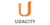# Introduction to Statistics

Level

## Introduction to StatisticsUdacity

Need more information? Get more details on the site of the provider.

### Starting dates and places

There are no known starting dates for this product.
• View related products with starting dates: Statistics.

## Making Decisions Based on Data

Statistics is about extracting meaning from data. In this class, we will introduce techniques for visualizing relationships in data and…

## Class Summary

Statistics is about extracting meaning from data. In this class, we will introduce techniques for visualizing relationships in data and systematic techniques for understanding the relationships using mathematics.

## What Should I Know?

This course does not require any previous knowledge of statistics. Basic familiarity with algebra such as knowing how to compute the mean, median and mode of a set of numbers will be helpful.

## What Will I Learn?

This course will cover visualization, probability, regression and ot…

There are no frequently asked questions yet. If you have any more questions or need help, contact our customer service.

## Making Decisions Based on Data

Statistics is about extracting meaning from data. In this class, we will introduce techniques for visualizing relationships in data and…

## Class Summary

Statistics is about extracting meaning from data. In this class, we will introduce techniques for visualizing relationships in data and systematic techniques for understanding the relationships using mathematics.

## What Should I Know?

This course does not require any previous knowledge of statistics. Basic familiarity with algebra such as knowing how to compute the mean, median and mode of a set of numbers will be helpful.

## What Will I Learn?

This course will cover visualization, probability, regression and other topics that will help you learn the basic methods of understanding data with statistics.

## Part 1: Visualizing relationships in data

Seeing relationships in data and predicting based on them; Simpson's paradox

## Part 2: Probability

Probability; Bayes Rule; Correlation vs. Causation

## Part 3: Estimation

Maximum Likelihood Estimation; Mean, Mean, Mode; Standard Deviation, Variance

## Part 4: Outliers and Normal Distribution

Outliers, Quartiles; Binomial Distribution; Central Limit Theorem; Manipulating Normal Distribution

## Part 5: Inference

Confidence intervals; Hypothesis Testing

## Part 6: Regression

Linear regression; correlation

## Course Instructors

Sebastian Thrun Instructor

Sebastian Thrun is a Research Professor of Computer Science at Stanford University, a Google Fellow, a member of the National Academy of Engineering and the German Academy of Sciences. Thrun is best known for his research in robotics and machine learning, specifically his work with self-driving cars.